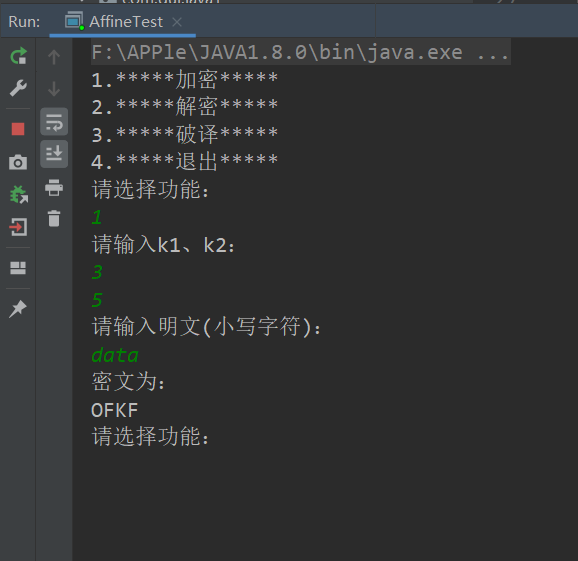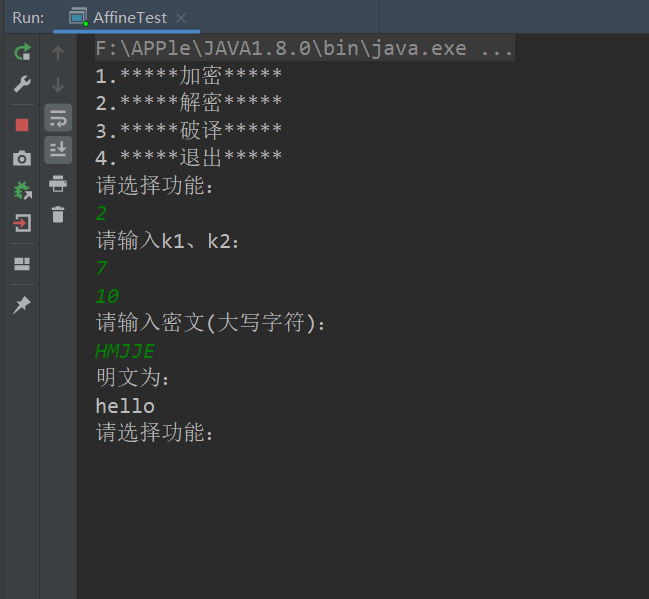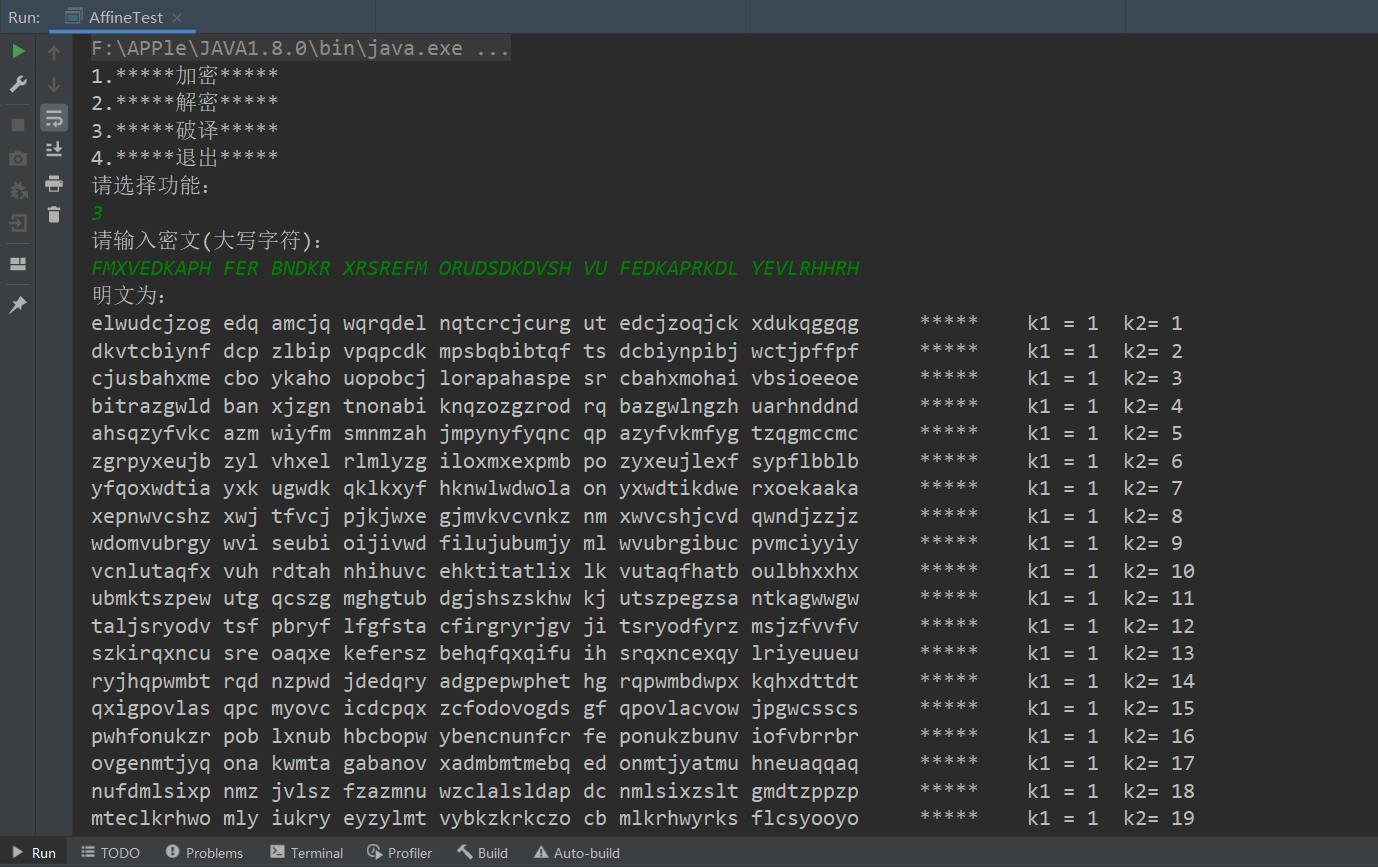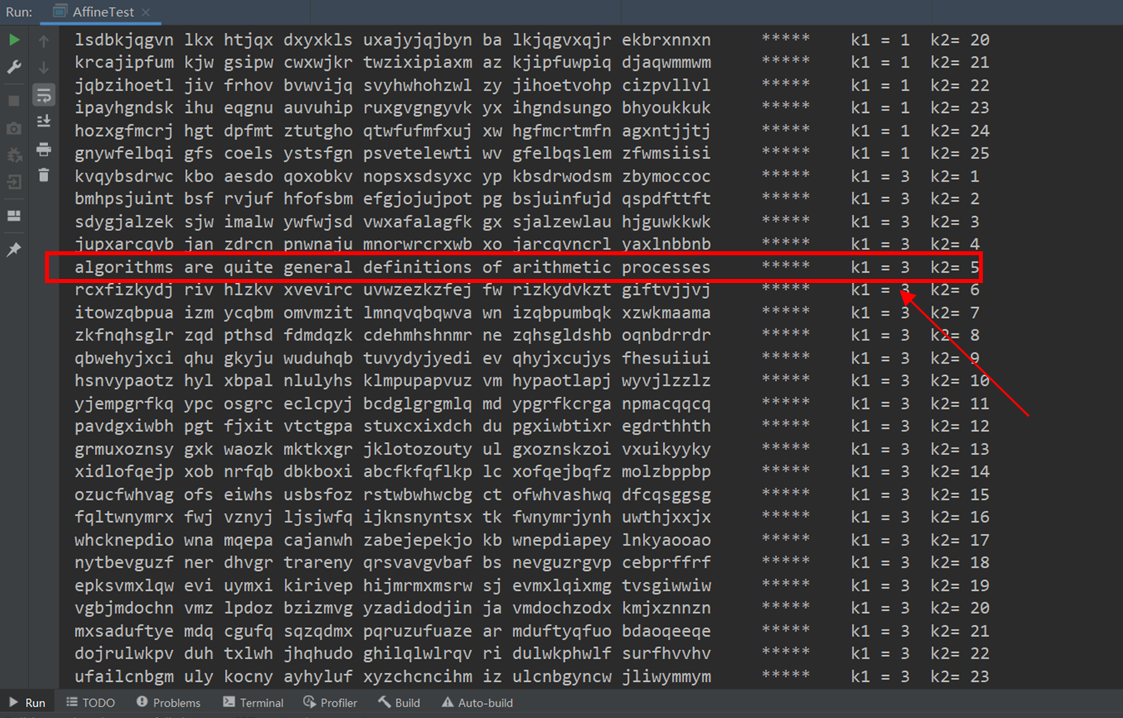• 仿射变换加密解密
千次阅读
2018-03-31 16:16:17

概述：基本上和数学上的仿射变换类似
y=ax+b，通过如此达到一一对应加密。

仿射变换加密加密过程：
加密算法：c=a*m+ b(mod n)

加密过程:
1.获取a，b（密钥），n（字符个数）

2.获取明文。
3.加密成密文，明文转换成各个字符所对应的数字，将所得数字带入上面的算法公式，得到数字再转换成对应的字符

解密过程：
算法：m=a^-1（m-b）（mod n）这里a^-1不是指倒数，而是a关于字符数量模的乘法可逆元，下面介绍一下乘法可逆元

乘法可逆元定义；
群G中任意一个元素a,都在G中有唯一的逆元a‘,具有性质aa'=a'a=e,其中e为群的单位元
这个官方的定义不学数论什么肯定看不太懂。。没事我们只研究数学应用，不学理论，

举个例子好了：
4关于模7的乘法逆元为多少?

可令4X≡1mod7，即可等价于4X=7K+1，其中X,K为整数，求X和K。
由此看出可逆元的通俗定义

如果ax≡1modf，那么a为关于模f的乘法逆元。
另外也有条件，当a与f互素时，a关于模f的乘法逆元有解。如果不互素，则无解。如果f为素数，则从1到f-1的任意数都与f互素，即在1到f-1之间都恰好有一个关于模f的乘法逆元。

再来一个更具体的例子，
求5关于14的乘法逆元

用辗转相除法
14=5*2+4

5=4*1+1
反过来写
1=5-4=5-（14-5*2）=5*3-14

因此5关于模14的乘法逆元为3.
如此即可求出逆元，然后再带入公式即可实现解密。

下面给出仿射加密算法的算法实现。（直观起见采用C++）

#include<iostream>
using namespace std;
#define N 26  //这里只采用26个字母的加解密

//加密
char *encry(char *Plain, int a, int b, int n);
char *decry(char *Cipher, int a, int b, int n);
//获取可行仿射加密的a值数组
void setArr(int Canuse[], int n);
//求GCD（最大公约数）
int Gcd(int a, int b);
//求a关于模n的乘法可逆元
int Multiplicative_inverse_modulo(int Canuse[], int a, int n);
int main() {
int a, b;
char str = "";
cout << ("输入密钥a，b的值") << endl;
cin >> a >> b;
cout << "输入明文内容" << endl;
cin >> str;
cout << "明文为" << endl;
cout << str << endl;
//加密
encry(str, a, b, N);
//输出密文
cout << str << endl;
//解密
decry(str, a, b, N);
//输出解密内容
cout << str << endl;
return 0;
}
//加密函数实现
char *encry(char *Plain, int a, int b, int n)
{
char *tmp = Plain;
if (Plain == NULL)return NULL;
while (*Plain) {
if (' ' == *Plain)
{
++Plain;
continue;
}
if ((*Plain < 'A') || (*Plain > 'Z'))
return NULL;
*Plain -= 'A';
*Plain = (a*(*Plain) + b) % n;
*Plain += 'A';
++Plain;
}
return tmp;
}
//解密所需基础算法实现
void setArr(int Canuse[], int n) {
for (int i = 1; i < n; i++) {
if (1 == Gcd(n, i))
*(Canuse++) = i;
}
}
int Gcd(int a, int b) {
int gcd = 0;
int div = 0;
//辗转相除法
do {
div = a%b;
gcd = b;
a = b;
b = div;
} while (div);
return gcd;
}
//求乘法可逆元
int Multiplicative_inverse_modulo(int Canuse[], int a, int n) {
for (int i = 0; Canuse[i] != 0; i++) {
if (1 == (a*Canuse[i]) % n)
return Canuse[i];
}
return 0;
}
//解密实现
char *decry(char *Cipher, int a, int b, int n) {
char *tmp = Cipher;
int Canuse = { 0 };//符合条件的a值
int moda = 0;//a的乘法可逆元
int i = 0;
if (Cipher == NULL)return NULL;
for (; i < 32; i++)Canuse[i] = 0;
setArr(Canuse, n);//存放符合条件的a.
moda = Multiplicative_inverse_modulo(Canuse, a, n);
while (*Cipher) {
if (' ' == *Cipher) {
++Cipher;
continue;
}
if ((*Cipher < 'A') || (*Cipher > 'Z'))
return NULL;
*Cipher -= 'A';
*Cipher = (moda*(*Cipher - b + n)) % n;
*Cipher += 'A';
++Cipher;
}
return tmp;
}
只恨数学学的不够多啊。MIMA
更多相关内容
• 1、加密 加密函数： E(m) = (k1 * m + k2)mod 26 ---->(以英文为载体，有26个字母） 注意：其中k1与26互为质数 2、解密 解密函数： D© = k1^-1(c - k2)(mod 26) —>k1^-1是k1的乘法逆元素 重点：求k1的乘法逆...

# 1、加密

### 加密函数：

E(m) = (k1 * m + k2)mod 26 ---->(以英文为载体，有26个字母）
注意：其中k1与26互为质数

# 2、解密

### 解密函数：

D© = k1^-1(c - k2)(mod 26)
—> k1^-1是k1的乘法逆元素
重点：求k1的乘法逆元素

乘法逆元素：要求在0,1,2,3,4，…，25找一个数，这个数和k1相乘再取模26运算，结果为1。

# 3、破译

要求：已知密文，求明文
未知：密钥 k1、k2
k1与26互为质数，且k1<26
故，k1可穷举，1,3,5,7,9,11,15,17,19,21,23,25
k2为正整数，且k2<26.
所以暴力破译后，有12*26 = 312 种情况

# Java代码实现：

package com.qul.java1;

import java.util.Scanner;

/**
* @author Dxkstart
* @create 2021-05-25 15:21
*/
public class AffineTest {
public static void main(String[] args) {

Scanner scanner = new Scanner(System.in);

System.out.println("1.*****加密*****");
System.out.println("2.*****解密*****");
System.out.println("3.*****破译*****");
System.out.println("4.*****退出*****");
boolean b = true;
while (b) {
System.out.println("请选择功能：");
int num = scanner.nextInt();

switch (num) {
case 1:
new Encryption().encryption();
break;
case 2:
new Decryption().decryption();
break;
case 3:
new Decode().decode();
break;
case 4:
b = false;
}
}

}

}

//加密算法
class Encryption {
String cleartext;//明文
int k1;//k1与26互为质数
int k2;

public void encryption() {
Scanner scanner = new Scanner(System.in);
scanner.useDelimiter("\n");

System.out.println("请输入k1、k2：");
k1 = scanner.nextInt();
k2 = scanner.nextInt();

System.out.println("请输入明文(小写字符)：");
cleartext = scanner.next();
System.out.println("密文为：");

char[] chars = cleartext.toCharArray();//String转char数组
for (int i = 0; i < chars.length; i++) {
int q = (int) chars[i];//转ACSll码 97-122
if (q != 32) {
int m = (k1 * (q - 97) + k2 % 26) % 26;
char c = (char) (m + 65);//转大写字符
System.out.print(c);
} else {
System.out.print(" ");
}
}
System.out.println();
}
}

//解密算法
class Decryption {
String ciphertext;//密文
int k1;//k1与26互为质数
int k2;

public void decryption() {
Scanner scanner = new Scanner(System.in);
scanner.useDelimiter("\n");//可识别空格字符

System.out.println("请输入k1、k2：");
k1 = scanner.nextInt();
k2 = scanner.nextInt();

System.out.println("请输入密文(大写字符)：");
ciphertext = scanner.next();
System.out.println("明文为：");

//求k1的乘法逆元素
int M;
for (M = 0; M < 26; M++) {
if ((k1 * M) % 26 == 1) {
break;
}
}

char[] chars = ciphertext.toCharArray();
for (int i = 0; i < chars.length; i++) {
int q = (int) chars[i];//转ACSll码 65-90
if (q != 32) {
int m = (M * ((q - 65 + 26) - k2)) % 26;
char c = (char) (m + 97);//转小写字符
System.out.print(c);
} else {
System.out.print(" ");
}
}
System.out.println();
}
}

//破译算法
class Decode {
String ciphertext;//密文
int k1;//k1与26互为质数
int k2;

public void decode() {
Scanner scanner = new Scanner(System.in);
scanner.useDelimiter("\n");

System.out.println("请输入密文(大写字符)：");
ciphertext = scanner.next();
System.out.println("明文为：");

//求k1、k2
for (k1 = 1; k1 <= 26; k1 += 2) {
if (k1 % 13 != 0) {
for (k2 = 1; k2 < 26; k2++) {
//求k1的乘法逆元素
int M;
for (M = 0; M < 26; M++) {
if ((k1 * M) % 26 == 1) {
break;
}
}

char[] chars = ciphertext.toCharArray();
for (int i = 0; i < chars.length; i++) {
int q = (int) chars[i];//转ACSll码 65-90
if (q != 32) {
int m = (M * ((q - 65 + 26) - k2)) % 26;
char c = (char) (m + 97);//转小写字符
System.out.print(c);
} else {
System.out.print(" ");
}
}
System.out.print("     *****    k1 = " + k1 + "  k2= " + k2);
System.out.println();
}
}

}

}

}



## 举例：

### 加密：### 解密：### 破译：

找出有真正意义的句子
破译结果：algorithms are quite general definitions of arithmetic processes
K1 = 3 k2 = 5展开全文java
• 用C写的仿射变换加密解密程序，程序过程很简单，不足之处，欢迎提出c++
• System.out.print("请输入你要加密的偏移量(k):"); t=input(); k=Integer.parseInt(t); System.out.print("请输入明码的倍乘因子(a)："); t=input(); a=Integer.parseInt(t); System.out.print( "请...
• 它是一个字母对一个字母的加密密码。定义明文空间P=Z26 P={\rm Z}_{26} P=Z26​ ，密文空间C=Z26 C={\rm Z}_{26} C=Z26​ ，秘钥空间为K={(a,b)∈Z26⋅Z26:gcd(a,26)=1} K=\lbrace (a,b)\in {\rm Z}_{26} \cdot {\rm...

仿射密码是一种古典移位密码，其算法设计时用到的数学基础是模运算和同余方程。它是一个字母对一个字母的加密密码。定义明文空间 P = Z 26 P={\rm Z}_{26} ，密文空间 C = Z 26 C={\rm Z}_{26} ，秘钥空间为 K = { ( a , b ) ∈ Z 26 ⋅ Z 26 : g c d ( a , 26 ) = 1 } K=\lbrace (a,b)\in {\rm Z}_{26} \cdot {\rm Z}_{26}:gcd(a,26)=1 \rbrace 对于 x ∈ P , y ∈ C , k = ( a , b ) ∈ K x\in P, y\in C,k=(a,b)\in K 定义加密函数 e k ( x ) = a x + b m o d      26 {\rm e}_k(x)=ax+b\mod \ 26
，定义解密函数 d k ( x ) = a − 1 ( y − b ) m o d      26 ) {\rm d}_k(x)=a^{-1}(y-b)\mod \ 26)
，其中是a在群的乘法逆元（求a在群的乘法逆元）。当a=1时，仿射密码，弱化为凯撒密码。

//加密
{
//开辟空间
for(int i=0;i<strlen(text);i++)
{
int code=text[i]-'a';
}
}


2.求逆过程: 拓展欧几里得算法

//求逆  b%m的逆
int extendedeuclid(int m,int b)
{
int a1,a2,a3;
int b1,b2,b3;
int t1,t2,t3;
a1=1;a2=0;a3=m;
b1=0;b2=1;b3=b;
while(1)
{
if(b3==0) return 0;
if(b3==1)
{
if(b2<0) b2=m+b2;
return b2;
}
int q=a3/b3;
t1=a1-q*b1;t2=a2-q*b2;t3=a3-q*b3;
a1=b1;a2=b2;a3=b3;
b1=t1;b2=t2;b3=t3;
}
return 0;
}


//解密
{
char* text=NULL;
text=(char*)malloc(10*sizeof(char));
{
text[i]=( (code-addkey+ 26) * extendedeuclid(26,mulkey) )% 26 + 'a';
}
return text;
}


4.完整代码：

#include <stdio.h>
#include <stdlib.h>
#include<string.h>

//求逆  b%m的逆
int extendedeuclid(int m,int b)
{
int a1,a2,a3;
int b1,b2,b3;
int t1,t2,t3;
a1=1;a2=0;a3=m;
b1=0;b2=1;b3=b;
while(1)
{
if(b3==0) return 0;
if(b3==1)
{
if(b2<0) b2=m+b2;
return b2;
}
int q=a3/b3;
t1=a1-q*b1;t2=a2-q*b2;t3=a3-q*b3;
a1=b1;a2=b2;a3=b3;
b1=t1;b2=t2;b3=t3;
}
return 0;
}

//加密
{
//开辟空间
for(int i=0;i<strlen(text);i++)
{
int code=text[i]-'a';
}
}

//解密
{
char* text=NULL;
text=(char*)malloc(10*sizeof(char));
{
text[i]=( (code-addkey+ 26) * extendedeuclid(26,mulkey) )% 26 + 'a';
}
return text;
}
int main()
{
char text;
printf("请输入明文:");
scanf("%s",text);
int k,b;
printf("请输入k,b的值:");
scanf("%d%d",&k,&b);
char *p=NULL;
char *q=NULL;
p=q=(char*)malloc(10*sizeof(char));
p=encode(text,k,b);
q=decode(p,k,b);
printf("加密后的密文:%s\n",p);
printf("解密后的明文:%s",q);
free(p);free(q);
return 0;
}



Cmd Markdown 公式指导手册
复习了一下C语言的指针，学的时候感觉难，现在看还是难。

展开全文c语言
• 前言：摘抄自wiki的关于仿射变换的定义：仿射变换，又称仿射映射，是指在几何中，一个向量空间进行一次线性变换并接上一个平移，变换为另一个向量空间。一个对向量平移，与旋转放大缩小的仿射映射为 【1】1、移位...

前言：

摘抄自wiki的关于仿射变换的定义：

仿射变换，又称仿射映射，是指在几何中，一个向量空间进行一次线性变换并接上一个平移，变换为另一个向量空间。

一个对向量平移，与旋转放大缩小的仿射映射为【1】

1、移位加密：

比方说：把字母表全部向右循环移1位，也就是A变成B，B变成C，... ，

Z变成A。

用数学一点的术语我觉得所谓“移位”就是映射吧。

那么我们可以写出通解公式，——现在是已知明文和加密步骤，进行加密，也就是求密文。

New = (Old + k) (mod table)

【2】

注释：

New：要求的密文

Old  ：明文

k ：移位的位数

table：这张表有多大，比方说字母表就是26个字母，table就是26.

2、仿射变换：

移位加密和仿射变换有啥关系呢？

额 ，因为移位加密就是一种放射变换。。。

首先我们在1里面的变量命名现在改一下，以便更好地认识，变成：

y

= (x + b)(mod m) 【3】

是不是和【1】很像啦，可惜我们的x没有系数。。

但是没关系，我们完全可以自己加上一个系数，把【3】变成：

y

= (ax + b)(mod m)   a,b为整数； 【4】

这样已经很像了，只不过【1】里面的自变量是向量。

但是，我们这里讨论的是一维的变换，所以不用用到2维及其以上的向量。(一维数字标记的向量就是普通的数嘛……)

3、知道了1、2这些，我们现在的目标就是——

"当知道一个一维的仿射变换的加密，

a. 怎么把明文加密

b.

怎么把密文解密 "

对于"a.

怎么把明文加密"，我们已经解决了，就是【4】那个公式。

对于"b.

怎么把密文解密"嘛，其实也不复杂。待我不快不慢地说来。

首先由【4】我们可以得到什么？

没错！就是 y

≡ (ax + b)(mod m) —— 这是明显的事实不用证明了。

然后正视一下题目是什么：我们现在已知a,b,y,m，要求x。

1). 左右移位一下 ,变为y-b

≡ (ax)(mod m) 【5】

{为啥【5】是对的？

首先同余式可以相加，

即 若 a ≡ b (mod m),c ≡ d (mod m)， 【*】

那么(a +

c) ≡ (b + d)(mod m) .

——同余式可以相加的证明用同余的定义就好……

然后  y ≡ (ax +

b)(mod m)

-b ≡ -b (mod

m)

所以……

}

2). 已知y-b

≡ (ax)(mod m)，x未知，求x.

现在已知ax ≡(y-b) (mod

m)；

{首先我们要知道 同余式可以相乘，若【*】，则 ac

≡ bd (mod m) .证明同样可以基于同余定义。}

这时候我们就脑补了，如果有一个c能使得

cax ≡ x ≡ c(y-b) (mod m)就好了！

也就是说这个c如果能使得ca = 1或者 更宽一点的：ca ≡ 1(mod

m).那么就解决了！

3). 于是问题变成了：找一个c使得，ca ≡ 1(mod

m)

于是很容易联系到费马小定理、欧拉定理一类的。

但是费马小定理要求m一定要是素数，这样和我们题目不符。

所以看看欧拉定理，对 gcd(a,m) =

1(m>1)，有aΦ(m)≡ 1 (mod m)

{Φ(m) 为欧拉函数，就是小于等于m的与m互素的正整数个数。如Φ(2) = 1

，Φ(6) = 2——6与{1,5}互素。}

所以如果ca

= aΦ(m)，那么就有ca ≡ 1(mod m)。

于是c

= aΦ(m)-1. 但是gcd(a,m) = 1，这一点不能漏。

4、综上，我们发现了，当c

= aΦ(m)-1时 ；

cax ≡c(y-b) (mod m)

化为 x ≡c(y-b) (mod m) {现在要附加gcd(a,m)=1这个条件了！}

5、那么对于仿射变换，

我们知道变化规则[即位移b]后，如何把密文[y]翻译成明文[x]呢？

结论是：

step

1.  求 c

= aΦ(m)-1

step 2.  x ≡c(y-b) (mod m)

番外小剧场：

窝：你在2里面为啥要改名字？该问题纯属好奇！

big窝：此中原因有2——

1. 如文中所说，为了更直观地比较。

2.

也是最根本的原因——变量命名如此之平凡，根本不是我的feel！OK？

窝：......

窝：第二个问题——你怎么知道同余式符合相加相乘原理？你怎么想到的？

big窝：

首先"同余式符合相加相乘原理"这个结论在数论里就像是实数有相加相乘的运算一样自然，如果想不到那么你需要找一本数论的书看看，不需要看完这就足以变成你的常识。至于怎么想到的，如果给你一堆数，你能想到的最基本的运算就是做加减乘除了吧？

窝：恩……好像是的……

big窝：那不就得了，说了同余式符合相加相乘原理是同余式的基本运算。

窝：……哦……

原文：http://www.cnblogs.com/PeanutPrince/p/3543723.html

展开全文• 加密解密都是数学函数。对此并没有很多了解。只为一解答一道作业题目来了解这两个函数以及将函数转为代码。 先从百度基本了解一下这个加密函数 只靠通识的数学知识，理解 是有难度 首先是计算优先级的疑惑...
•python
• 基于matlab的图像加密处理，主要用到仿射变换，双随机相位处理等。对图像进行加密和还原。
• 这个是几年前《密码学》课程设计时做的东西了， 给各位参考。
• ## 密码学之仿射加密解密算法

万次阅读 多人点赞 2017-03-09 15:38:04
仿射变换加密解密分别是： c = Ea,b(m) ≡ a, + b(mod 26) m = Da,b(c) ≡ a^-1(c - b)(mod 26) 其中，a,b是密钥，为满足0≤a,b≤25和gcd(a,26)等于1的整数。 其中gcd(a,26)表示a和26的最大公因子，gcd(a,26)=...算法
• 目录简介加密函数解密函数乘法逆元例题加密解密：代码（python版） 简介 仿射密码为单表加密的一种，字母系统中所有字母都藉一简单数学方程加密，对应至数值，或转回字母。它是一种替换密码，利用加密函数一个字母...python
• ## Java实现仿射密码加密解密

千次阅读 热门讨论 2019-01-17 13:49:36
Java实现仿射密码加密解密 仿射密码 加密：将明文转化为对应的数字，如 ‘a’-&gt; 0, ‘b’-&gt;1,…,’1’-&gt;26,’2’-&gt;27,…然后将数字进行仿射运算，求取出来的数字再转化为字符。即 密文=...
• 仿射加密算法的C实现，本人写的代码。加密解密可以重复进行。
• 仿射变换加密 多表代换加密 例题：仿射变换 例题：多表代换 古典密码简介 古典密码主要有置换和代换的方法。置换：字母重新排列，字母本身不变，但其位置改变了（凯撒密码、移位变换）。代换：将明文中的字符...python 算法
• 仿射变换解密原理 仿射变换是基于凯撒密码（替换）和移位变换，需要两个密钥，一个为相乘a，一个为移位b。 加密算法：c=a*m+ b(mod n) 加密过程: 1.获取a，b（密钥），n（字符个数），mod n是为了保证得到数字唯一...
• 仿射密码为单表加密的一种，字母系统中所有字母都藉一简单数学方程加密，对应至数值，或转回字母mima
• 代换密码算法的原理是使用替代法进行加密，就是将明文中的字符用其它字符替代后形成密文。... name:仿射变换解密 author:Lsy date:2020-11-18 } ''' # 加密函数 def encrypt(Plaintext, a, b):
• 加密程序 解密程序 待加密文本 待解密文本 加密函数: Y=（AX+B）%26 解密函数: X=（A的逆元）*（Y-B）%26 加密程序 #include&lt;stdio.h&gt; int main(){ FILE *fp = ("fangshe1.txt"...
• 基于matlab仿射变换数字图象置乱 二、源代码 function chengxu() A=imread('lena.png'); figure,imshow(A); title('原图像(256*256)'); for K=1:16 %置乱16次 figure,imshow(B); title(['置乱',num2str(K),'次...matlab 自动驾驶 开发语言
• 实现仿射变换加密解密算法，例程实现对任意26个英文字母的加密，需选定k1和k2。
• 根据下图仿射密码（变换）加解密的描述，用所熟悉的语言，完成实验内容、描述实验操作步骤、实验结果与实验心得。 实验环境 计算机语言：Python 开发环境：Pycharm 原理 实验内容 编程实现仿射密码，要求有加密...python
• 本实验为华中科技大学计算机学院信息安全专业的“信息系统安全”课程实验之一。文件包含有三大古典加密解密技术的实现代码（C#）以及实验说明性文档！！！
• 1、用仿射变换3x+5加密如下明文：data 2、用仿射变换7x+10解密如下密文：HMJJE 3、用仿射变换破译如下密文： FMXVEDKAPHFERBNDKRXRSREFMORUDSDKDVSHVUFEDKAPRKDLYEVLRHHRH #include #include<string.h> using ...
• 程序简介：本程序实现了仿射加密算法，密钥1须与26互素，密钥2可为任意整数 密文和明文空间：a-z,A-Z; 程序优点：1，算法实现条理清楚；2，可按菜单重复进行加密解密 程序缺点：1，使用了全局变量
• 该压缩包包含了Python脚本编写的摩斯密码加密解密、培根密码加密解密、ASCII编码解码、凯撒密码加密解密，可用于教学和实操...